## 并查集笔记

Published: 12 May 2012 Category:

1.图示非常丰富，阅读起来更有趣，更易懂；
2.给出了测试算法用的不同规模的数据和测试方法，以及展示算法结果所需要的工具。这些工具使得算法的测试、结果展示非常方便、直观。
3.介绍问题时更自然，相比算法导论定义、证明的方式，本书给出算法的应用场景，对需要解决的问题，不断改进算法得到最优结果，逐步改进的思路符合人们研究问题的正常过程，思维不跳跃，使学习过程有逻辑可循。

</br>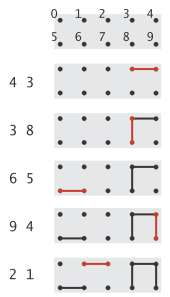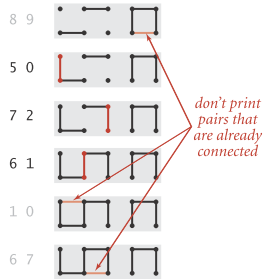1.输入的整数代表网络中的计算机，数对代表网络中的连接。我们的程序用来判断为了让p和q通信，是建立一个新的直接连接，还是可以用已有的连接建立通信路径；
2.整数代表电子电路中的连接点，数对代表连接各点的线路；
3.整数代表社交网络中的人，数对代表好友关系。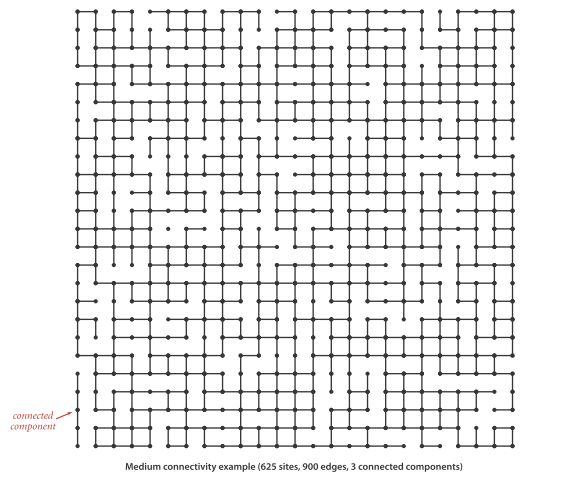[code lang=”java”] //Union-find API public class UF UF(int N) //initialize N sites with integer names (0 to N-1) void union(int p, int q) //add connection between p and q int find(int p) //component identifier for p (0 to N-1) boolean connected(int p, int q) //return true if p and q are in the same component int count()// number of components [/code]

[code lang=”java”] public class UF{ private int[] id; //组件标识数组 private int count; //组件数量

``````public UF(int N){
id = new int[N];
for(int i = 0; i &lt; N; i++)
id[i] = i;
count = N;
}

public void union(int p, int q){}
public int find(int p){}

public boolean connected(int p, int q){
return find(p) == find(q);
}

public int count(){
return count;
}

public static void main(String[] args){
int N = StdIn.readInt();
UF uf = new UF(N);
while(!StdIn.isEmpty()){
int p = StdIn.readInt();
int q = StdIn.readInt();
if(uf.connected(p, q)) continue;
uf.union(p, q);
Stdout.println(p + &quot; &quot; + q);
}
} } [/code]
``````

UF类中提供了供测试用的main方法，它读取输入的整数对，如果数对没有连通，就将其连通并输出此数对。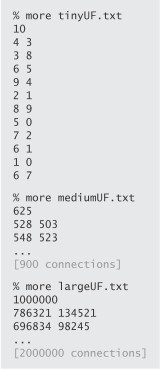（一）Quick-find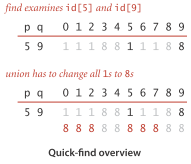[code lang=”java”] pubic int find(int p){ return id[p]; }

public void union(int q){ int pID = find(p); int qId = find(q); if(pID == qID) return; for(int i = 0; i < id.length; i++){ if(id[i] == pID) id[i] = qID; } count–; } [/code]

find()操作很快，因为每次操作只访问id[]一次。但是union()操作每次都需要遍历id[]一遍，因此quick-find算法对大数据量问题不适用。
quick-find算法每次find()操作需一次数组访问，每次union()需要N+3到2N+1次数组访问（每次union()，需要两次find()，测试id[]中所有项，并更改其中1到N-1个项）。

（二）Quick-union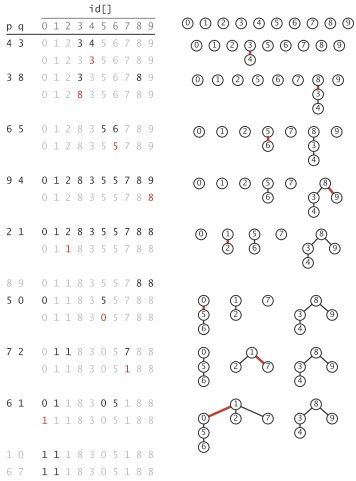quick-union算法的id[]实际上实现了树的森林表示，id[]项是指向父节点的链接，根节点有指向自身的链接。森林中的每棵树是一个组件，根为该组件的标识。

[code lang=”java”] pubic int find(int p){ while(id[p] != p) p = id[p]; return p; } public void union(int q){ int pRoot = find(p); int qRoot = find(q); if(pRoot == qRoot) return; id[pRoot] = qRoot; count–; } [/code]

quick-union似乎比quick-find快速，因为对每个输入数对，它不需要遍历整个数组，但是具体快了多少？在最优情况下，find()只需要一次数组访问获得组件标识；最坏情况下，find()需要2N+1次数组访问。具体性能依赖于输入的情况。我们在此不进行quick-find和quick-union的比较分析，因为接下来会研究一个更好的算法。在此可以认为，quick-uinon比quick-find有所提高，因为quick-find的union操作总需要线性时间，而quick-union并不总需要（取决于输入）。
find()操作的数组访问为1加上当前点的2倍深度；union()和connected()的数组访问次数是两个find()操作（如两个点属于不同树，union操作需要再加1）。

（三）Weighted quick-union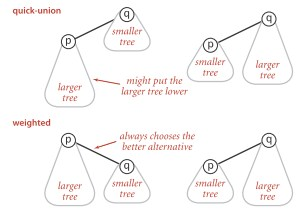[code lang=”java”] public class WeightedQuickUnionUF { private int[] id; private int[] sz; private int count;

``````public WeightedQuickUnionUF(int N){
count = N;
id = new int[N];
sz = new int[N];
for(int i = 0; i &lt; N; i++){
id[i] = i;
sz[i] = 1;
}
}

public int count(){
return count;
}

public boolean connected(int p, int q){
return find(p) == find(q);
}

private int find(int p){
while(p != id[p]) p = id[p];
return p;
}

public void union(int p , int q){
int i = find(p);
int j = find(q);
if(i == j) return;
if(sz[i] &lt; sz[j]){
id[i] = j;
sz[j] += sz[i];
}
else{
id[j] = i;
sz[i] += sz[j];
}
count--;
} } [/code]
``````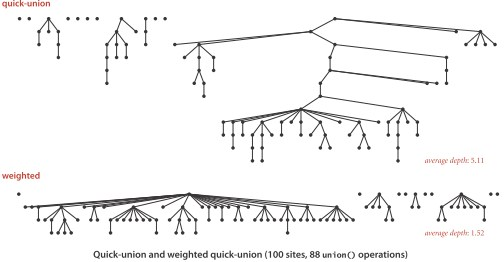算法 union find quick-find N 1 quick-union tree height tree height weighted quick-union lgN lgN impossible 1 1

Algorithms一书更容易让人体会到学习、使用算法的快乐之处。如果你在《算法导论》的数学证明中有些疲倦时，不妨找来这本书，重拾初学者的乐趣。

#### 简介Happier.